UPSC  >  Exercise 11.1 NCERT Solutions - Constructions

# Exercise 11.1 NCERT Solutions - Constructions - NCERT Textbooks (Class 6 to Class 12) - UPSC

## Basic Constructions

A geometrical construction means to draw geometrical figures, such as an angle, a circle, a triangle, a quadrilateral, and a polygon, etc.
We normally use all or some of the following instruments for drawing geometrical figures: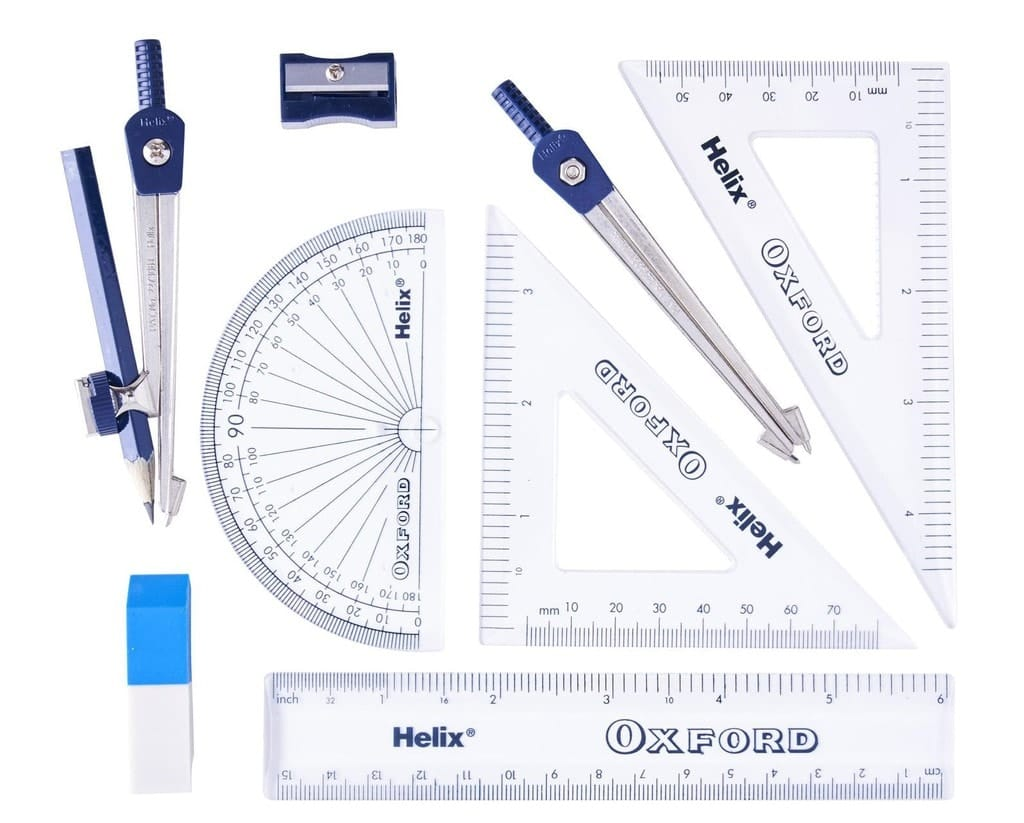• A protractor
• A pair of compasses
• A pair of set squares
• A pair of dividers

## Exercise 11.1

Q1. Construct an angle of 90º at the initial point of a given ray and justify the construction.
Ans: Steps of construction:

• Draw a ray OA.
• Taking O as the centre and suitable radius, draw a semicircle, which cuts OA at B.
• Keeping the radius the same, divide the semicircle into three equal parts such that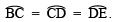• Draw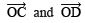• Draw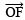, the bisector of ∠COD.

Thus, ∠AOF = 90º.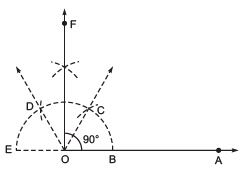Justification:

∵ O is the centre of the semicircle and it is divided into 3 equal parts.∠BOC = ∠COD = ∠DOE
∵ Equal chords subtend equal angles at the centre
∴ ∠BOC + ∠COD + ∠DOE = 180º
∠BOC + ∠BOC + ∠BOC = 180º
3∠BOC = 180°
∴ ∠BOC = 60º
Similarly, ∠COD = 60º and ∠DOE = 60º
∵ OF is the bisector of ∠COD.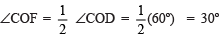= 30º
Now, ∠BOC + ∠COF = 60º + 30º
∠BOF = 90º or ∠AOF = 90º

Q2. Construct an angle of 45º at the initial point of a given ray and justify the construction.
Ans: Steps of construction:

• Draw a ray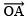.
• Taking O as the centre and with a suitable radius, draw a semicircle such that it intersectsat B.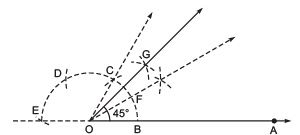• Taking B as centre and keeping the same radius, cut the semicircle at C. Similarly, cut the semicircle at D and E, such that. Join OC and produce.
• Divide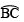into two equal parts, such that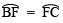• Draw OG, the angle bisector of ∠FOC.

Thus, ∠BOG = 45º or ∠AOG = 45º
Justification:∴ ∠BOC = ∠COD = ∠DOE
∵ Equal chords subtend equal angles at the centre
∴ ∠BOC + ∠COD + ∠DOE = 180º
∠BOC = 60º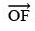is the bisector of  ∠BOC/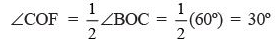.. (1)
Also,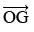is the bisector of  ∠COF.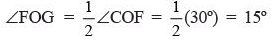... (2)
Adding (1) and (2), we get∠COF + ∠FOG = 30º + 15º = 45º
∠BOF + ∠FOG = 45º   [∵ ∠COF = ∠BOF]
∠BOG = 45º

Q3. Construct the angles of the following measurements:
(a) 30º
(b)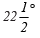(c) 15º
Ans:

(a) Angle of 30º
Steps of construction:

• Draw a ray OA.
• With O as the centre and a suitable radius, draw an arc, cutting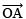at B.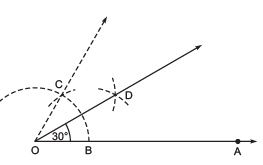• With centre at B and the same radius as above, draw an arc to cut the previous arc at C.
• Join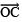and produce, such that ∠BOC = 60º.
•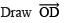bisector of ∠BOC, such that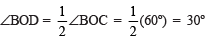Thus, ∠BOD = 30º
(b) Angle of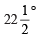Steps of construction:

• Draw a ray• Draw an angle ∠AOB = 90º
• Draw OC, the bisector of ∠AOB, such that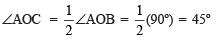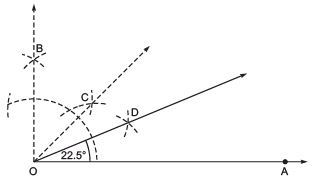• Now, draw OD, the bisector of ∠AOC, such that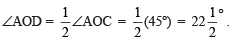Thus,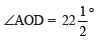(c) Angle of 15º
Steps of construction:

• Draw a ray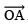.
• Construct ∠AOB = 60º.
• Draw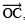the bisector of ∠AOB, such that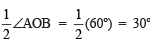i.e. ∠AOC = 30º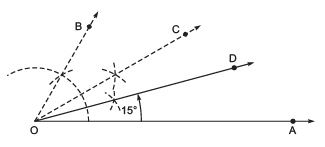• Draw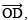the angle bisector of ∠AOC such that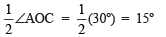Thus, ∠AOD = 15º

Q4. Construct the following angles and verify by measuring them by a protractor:
(a) 75º
(b) 105º
(c) 135º
Ans:

(a) Angle of 75º (Hint: 75º = 60º + 15º)
Steps of construction:

• Draw.
• With O as centre and having a suitable radius, draw an arc which meets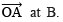• With centre B and keeping the radius same, mark a point C on the previous arc.
• With centre C and the same radius, mark another point D on the arc of step 2.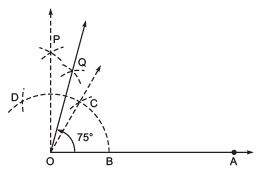• Draw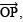the bisector of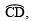such that ∠COP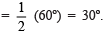• Draw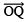, the bisector of ∠COP, such that ∠COQ = 15º

Thus, ∠BOQ = 60º + 15º = 75ºor ∠AOQ = 75º.

(b) Angle of 105º (Hint: 105º = 90º + 15º)

Steps of construction:

• Draw• With centre O and having a suitable radius, draw an arc that meets OA at B.
• With centre B and keeping the same radius, mark a point C on the arc of step 2.
• With centre C and keeping the same radius, mark another point D on the arc of step 2.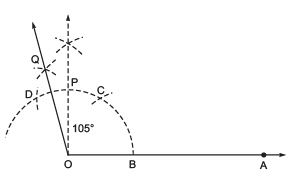•  Draw OP, the bisector of• Draw OQ, the bisector of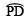.

Thus, ∠AOQ = 105º

(c) Angle of 135º (Hint: 120º + 15º = 135º)
Steps of construction:

• Draw a ray• With centre O and having a suitable radius draw an arc to meet OP at A.
• Keeping the same radius and starting from A, mark points Q, R and S on the arc of step 2.
• Draw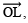the bisector of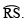V. Draw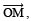the bisector of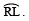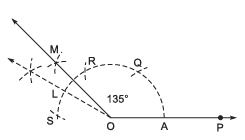Thus, ∠POM = 135º.

Q5. Construct an equilateral triangle, given its side and justify the construction.
Ans: Let us construct an equilateral triangle, each of whose side = PQ
Steps of construction:

• Draw a ray.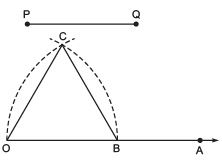• Taking O as centre and radius equal to PQ, draw an arc to cut OA at B such that OB = PQ
• Taking B as centre and radius = OB, draw an arc, to intersect the previous arc at C.
• Join OC and OB.

Thus, ΔOBC is the required equilateral triangle.
Justification:
∵ The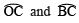are drawn with the same radius.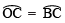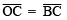∵ Chords corresponding to equal arcs are equal.
∵ OC = OB = BC
∴ ΔOBC is an equilateral triangle.

The document Exercise 11.1 NCERT Solutions - Constructions | NCERT Textbooks (Class 6 to Class 12) - UPSC is a part of the UPSC Course NCERT Textbooks (Class 6 to Class 12).
All you need of UPSC at this link: UPSC

## NCERT Textbooks (Class 6 to Class 12)

1402 docs|679 tests

## FAQs on Exercise 11.1 NCERT Solutions - Constructions - NCERT Textbooks (Class 6 to Class 12) - UPSC

 1. What are the basic constructions in geometry?Ans. The basic constructions in geometry include constructing a perpendicular bisector of a line segment, constructing an angle bisector, constructing a perpendicular from a point to a line, constructing parallel lines, and constructing triangles of different types.
 2. How do you construct a perpendicular bisector of a line segment?Ans. To construct a perpendicular bisector of a line segment, we follow these steps: 1. Draw an arc from each endpoint of the line segment. 2. From the intersections of the arcs, draw two more arcs that intersect each other. 3. Draw a straight line connecting the midpoint of the line segment to the intersection of the two arcs. This line is the perpendicular bisector.
 3. What is the purpose of constructing an angle bisector?Ans. The purpose of constructing an angle bisector is to divide an angle into two equal parts. This construction is often used in various geometric problems and proofs.
 4. How can you construct a perpendicular from a point to a line?Ans. To construct a perpendicular from a point to a line, follow these steps: 1. Draw a line segment from the given point to any point on the line. 2. On this line segment, construct the perpendicular bisector. 3. The intersection of the perpendicular bisector with the given line is the desired perpendicular from the point to the line.
 5. Can you explain how to construct parallel lines?Ans. Yes, to construct parallel lines, follow these steps: 1. Draw a line segment and a point outside the line segment. 2. From the given point, construct a perpendicular to the line segment. 3. Using the same length as the line segment, draw an arc from the endpoint of the line segment. 4. From the other endpoint, draw an arc intersecting the first arc. 5. Draw a line connecting the intersection points of the arcs. This line is parallel to the given line segment.

## NCERT Textbooks (Class 6 to Class 12)

1402 docs|679 testsExplore Courses for UPSC exam### How to Prepare for UPSC

Read our guide to prepare for UPSC which is created by Toppers & the best Teachers
Signup to see your scores go up within 7 days! Learn & Practice with 1000+ FREE Notes, Videos & Tests.
10M+ students study on EduRev
Track your progress, build streaks, highlight & save important lessons and more!
Related Searches

,

,

,

,

,

,

,

,

,

,

,

,

,

,

,

,

,

,

,

,

,

;